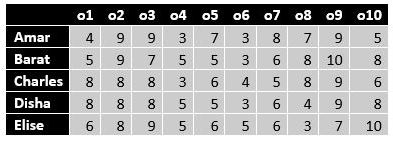# workshop

Set 1:

7 runners with vest numbers 1 to 7 participated in a race and finished the race with no ties.  The following has been observed after the race has been finished.

• The square of the highest total of the vest number and rank is 169 which occurs only once.
• The square of the lowest total of vest number and rank is 16 and occurs only once
• The square of the total of vest number and rank = 81, occurs thrice.
• The winner's vest number exceeds that of the first runner-up.
1. Vest number of sprinter coming first is:

a. 3
b. 6
c. 1
d. 5

2. Vest number of sprinter coming Second is:
a. 7
b. 6
c. 4
d. 3

3. Vest number of sprinter finishing third is:
a. 6
b. 3
c. 1
d. 2

Set 2:
Six people call them - A, B, C, D, E and F – were discussing the scores made by India’s top five batsmen in a match. They made the following statements regarding the scores of the five batsmen.
A - Exactly four of them scored a total of 180 runs.
B - Exactly four of them scored a total of 150 runs.
C - Exactly four of them scored a total of 130 runs.
D - Exactly four of them scored a total of 170 runs.
E - Exactly four of them scored a total of 160 runs.
F - Exactly four of them scored a total of 140 runs.

It was later observed that only one of them had done a mistake while adding the scores.

4. What can be the highest score made by any batsman in the match?
5. Using the information given, scores of how many batsmen can be exactly determined?
6. If one of the batsmen scored 30 runs, then what is the total score of all the five batsmen?
a. 195
b. 190
c. 200
d. CBD

7. If the sum of the scores of exactly two batsmen is 40, then which of the following persons definitely did not make a mistake while adding the scores?
a. D
b. C
c. B
d. Cannot be determined

8. What could be the maximum possible total scored by these five batsmen together?

Set3:
Ten objects o1, o2, …, o10 were distributed among Amar, Barat, Charles, Disha, and Elise. Each item went to exactly one person. Each person got exactly two of the items, and this pair of objects is called her/his bundle.

The following table shows how each person values each object.The value of any bundle by a person is the sum of that person's values of the objects in that bundle. A person X envies another person Y if X values Y's bundle more than X's own bundle.

For example, hypothetically suppose Amar's bundle consists of o1 and o2, and Barat's bundle consists of o3 and o4. Then Amar values his own bundle at 4 + 9 = 13 and Barat's bundle at 9 + 3 = 12. Hence Amar does not envy Barat. On the other hand, Barat values his own bundle at 7 + 5 = 12 and Amar's bundle at 5 + 9 = 14. Hence Barat envies Amar.

The following facts are known about the actual distribution of the objects among the five people.
1. If someone's value for an object is 10, then she/he received that object.
2. Objects o1, o2, and o3 were given to three different people.
3. Objects o1 and o8 were given to different people.
4. Three people value their own bundles at 16. No one values her/his own bundle at a number higher than 16.
5. Disha values her own bundle at an odd number. All others value their own bundles at an even number.
6. Some people who value their own bundles less than 16 envy some other people who value their own bundle at 16. No one else envies others.

9. What BEST can be said about object o8?
a. o8 was given to Disha
b. o8 was given to Amar, Charles, or Disha
c. o8 was given to Charles or Disha
d. o8 was given to Charles

10. Who among the following envies someone else?
a. Amar
b. Barat
c. Charles
d. Elise

11. What is Amar's value for his own bundle?

12. Object o4 was given to
a. Disha
b. Barat
c. Charles
d. Elise

13. Object o5 was given to
a. Amar
b. Elise
c. Charles
d. Disha

14. What BEST can be said about the distribution of object o1?
a. o1 was given to Charles or Disha
b. o1 was given to Charles, Disha, or Elise
c. o1 was given to Charles
d. o1 was given to Disha